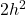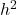## A parallelograms base is twice its height. If the parallelogram has an area of 32 square feet, what are the lengths og the base and height.​

Question

A parallelograms base is twice its height. If the parallelogram has an area of 32 square feet, what are the lengths og the base and height.​

in progress 0
3 months 2021-08-15T08:03:43+00:00 1 Answers 1 views 0

4 ft. = height

8 ft. = base

Step-by-step explanation:

Let h = length of the height

2h = length of the base

A = bh

32 = 2h(h) == 16

h = 4 ft. = height

8 ft. = base